Semiconductor Types

# Semiconductor Types - Notes | Study Analog Electronics - Electrical Engineering (EE)

 Table of contentsClassification of Semiconductors1. Intrinsic Semiconductors2. Extrinsic SemiconductorDifference between Intrinsic and Extrinsic semiconductors:1 Crore+ students have signed up on EduRev. Have you?

Classification of Semiconductors
Semiconductors can be classified as:

1. Intrinsic Semiconductor
2. Extrinsic Semiconductor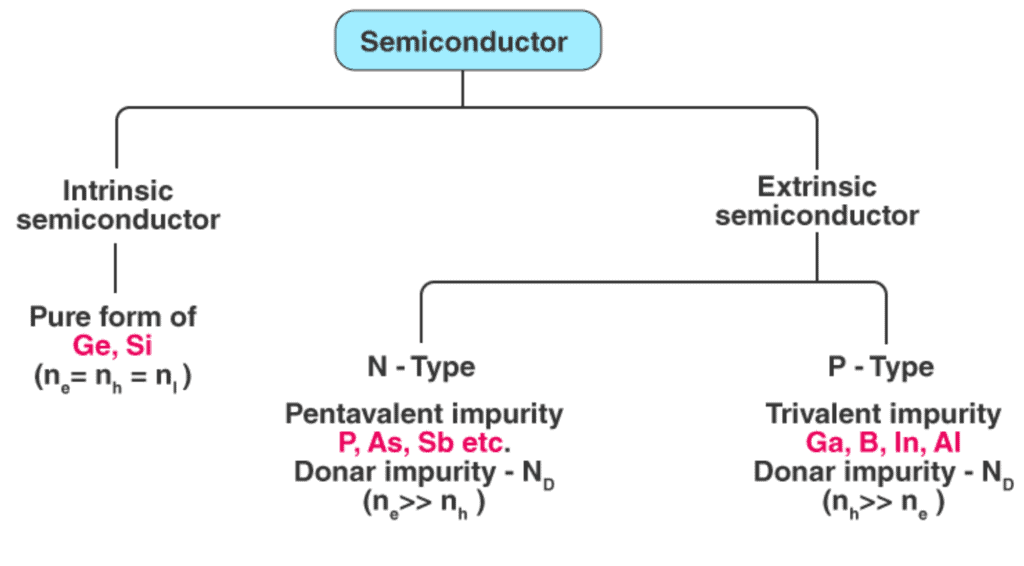1. Intrinsic Semiconductors

• A pure form of semiconductor is called an intrinsic semiconductor. Conduction in an intrinsic semiconductors is either due to thermal excitation or crystal defects. Si and Ge are the two most important semiconductors used. Other examples include Gallium arsenide (GaAs), Indium Antimonide (InSb) etc.
• Let us consider the structure of Si. The atomic no. of Si is 14 and it has 4 valence electrons. These 4 electrons are shared by four neighbouring atoms in the crystal structure by means of covalent bond.
• Fig. 1.2a shows the crystal structure of Si at absolute zero temperature (0K). Hence a pure semiconductor has poor conductivity (due to lack of free electrons) at low or absolute zero temperature.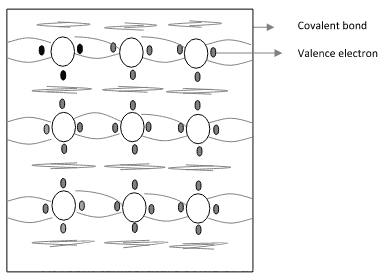Fig. 1.2a Crystal structure of Si at 0k
• In fig.1.2b the valence electrons that jump into conduction band are called free electrons that are available for conduction.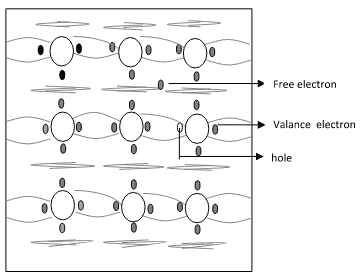Fig. 1.2b Crystal Structure of Si at Room Temperature 0k

• The absence of electrons in covalent bond is represented by a small circle usually referred to as hole which is of positive charge. Even a hole serves as carrier of electricity in a manner similar to that of a free electron.

The mechanism by which a hole contributes to conductivity is explained as follows:

• When a bond is incomplete so that a hole exists, it is relatively easy for a valence electron in the neighbouring atom to leave its covalent bond to fill this hole. An electron moving from a bond to fill a hole moves in a direction opposite to that of the electron.
• This hole, in its new position, may now be filled by an electron from another covalent bond and the hole will correspondingly move one more step in the direction opposite to the motion of electron. Here we have a mechanism for conduction of electricity which does not involve free electrons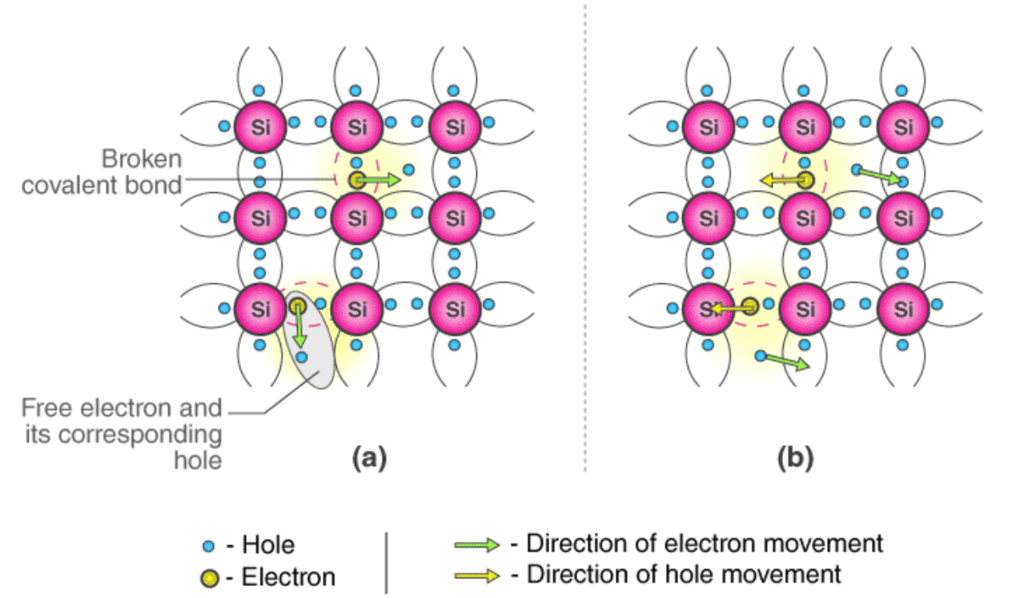Conduction Mechanism in Case of Intrinsic Semiconductors (a) In absence of Electric Field (b) In presence of Electric Field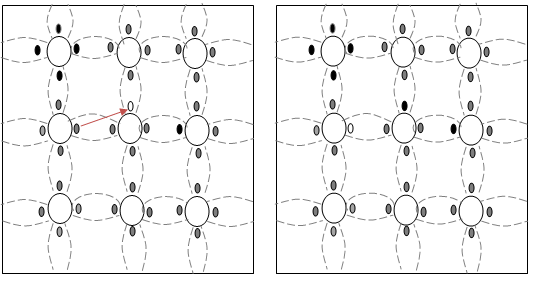Fig 1.3 (a) & 1.3 (b)

• Fig 1.3a show that there is a hole at ion 6. Imagine that an electron from ion 5 moves into the hole at ion 6 so that the configuration of 1.3b results. If we compare both fig1.3a & fig 1.3b, it appears as if the hole has moved towards the left from ion 6 to ion 5. Further, if we compare fig 1.3b and fig 1.3c, the hole moves from ion 5 to ion 4.
• This discussion indicates that the motion of hole is in a direction opposite to that of motion of electron. Hence, we consider holes as physical entities whose movement constitutes flow of current.
• In a pure semiconductor, the number of holes is equal to the number of free electrons.

The Lattice of Pure Silicon Semiconductor at Different Temperatures

• At absolute zero Kelvin temperature: At this temperature, the covalent bonds are very strong and there are no free electrons and the semiconductor behaves as a perfect insulator.
• Above absolute temperature: With the increase in temperature few valence electrons jump into the conduction band and hence it behaves like a poor conductor.

Energy Band Diagram of Intrinsic Semiconductor

The energy band diagram of an intrinsic semiconductor is shown below: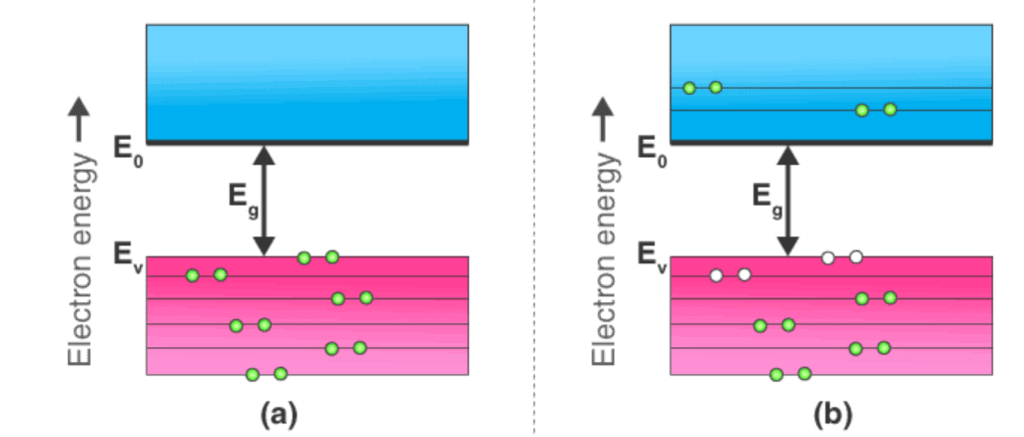In intrinsic semiconductors, current flows due to the motion of free electrons as well as holes. The total current is the sum of the electron current Ie due to thermally generated electrons and the hole current IhTotal Current (I) = Ie + IhFor an intrinsic semiconductor, at finite temperature, the probability of electrons to exist in conduction band decreases exponentially with increasing bandgap (Eg)n = n0e-Eg/2.Kb.where,

• Eg = Energy bandgap
• Kb = Boltzmann’s constant

Question for Semiconductor Types
Try yourself:Semi conductor in pure form is ______ semiconductor.

2. Extrinsic Semiconductor

• Intrinsic semiconductor has very limited applications as they conduct very small amount of current at room temperature.
• The current conduction capability of intrinsic semiconductor can be increased significantly by adding small amounts of impurity to the intrinsic semiconductor.
• By adding impurities, it becomes impure or extrinsic semiconductor. This process of adding impurities is known as doping. The amount of impurity added is 1 part in 106 atoms.

(i) N-type Semiconductor

• If the added impurity is a pentavalent atom then the resultant semiconductor is called N-type semiconductor. Examples of pentavalent impurities are Phosphorus, Arsenic, Bismuth, Antimony etc.
• A pentavalent impurity has five valence electrons. Fig 1.3a shows the crystal structure of N-type semiconductor material where four out of five valence electrons of the impurity atom(antimony) forms covalent bond with the four intrinsic semiconductor atoms. The fifth electron is loosely bound to the impurity atom.
• This loosely bound electron can be easily excited  from the valence band to the conduction band by the application of electric field or increasing the thermal energy. The energy required to detach the fifth electron form the impurity atom is very small of the order of 0.01eV for Ge and 0.05 eV for Si.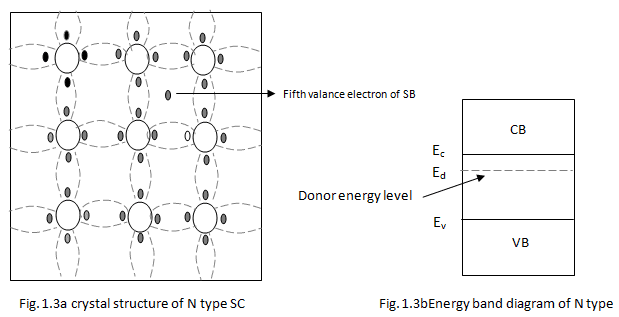• The effect of doping creates a discrete energy level called donor energy level in the forbidden band gap with energy level Ed slightly less than the conduction band (fig 1.3b).
• The difference between the energy levels of the conducting band and the donor energy level is the energy required to free the fifth valence electron (0.01 eV for Ge and 0.05 eV for Si).
• At room temperature, almost all the electrons from the donor impurity atom are raised to conduction band and hence the number of electrons in the conduction band increases significantly. Thus, every antimony atom contributes to one conduction electron without creating a hole.
• In the N-type semiconductor, the no. of electrons increases and the no. of holes decreases compared to those available in an intrinsic semiconductor. The reason for decrease is the no. of holes is that the larger no. of electrons present increases the recombination of electrons with holes.
• Thus, current in N-type semiconductor is dominated by electrons which are referred to as majority carriers. Holes are the minority carriers in N-type semiconductor.

(ii) P-type Semiconductor

• If the added impurity is a trivalent atom, then the resultant semiconductor is called P-type semiconductor. Examples of trivalent impurities are Boron, Gallium, indium etc.
• The crystal structure of  P-type semiconductor is shown in the fig1.3c. The three valence electrons of the impurity (boron) forms three covalent bonds with the neighboring atoms and a vacancy exists in the fourth bond giving rise to holes.
• The hole is ready to accept an electron from the neighbouring atoms. Each trivalent atom contributes to one hole generation and thus introduces a large no. of holes in the valence band.
• At the same time, the no. of electrons are decreased compared to those available in an intrinsic semiconductor because of increased recombination of holes and electrons.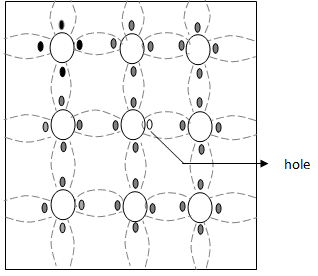Fig. 1.3c Crystal structure of P-type Semiconductor
• Thus, in a P-type semiconductor, holes are majority carriers and electrons are minority carriers. Since each trivalent impurity atom is capable of accepting an electron, these are called acceptor atoms. The following fig 1.3d shows the pictorial representation of P-type semiconductor.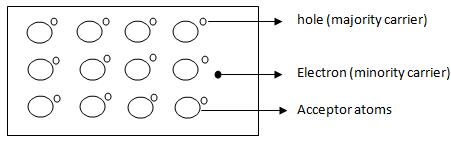Crystal Structure of P-type Semiconductor

• The conductivity of N-type semiconductor is greater than that of P-type semiconductor as the mobility of electron is greater than that of hole.
• For the same level of doping in N-type semiconductor and P-type semiconductor, the conductivity of an N-type semiconductor is around twice that of a P-type semiconductor.

Question for Semiconductor Types
Try yourself:In P-type semiconductor, the majority and minority charge carriers are respectively

Difference between Intrinsic and Extrinsic semiconductors: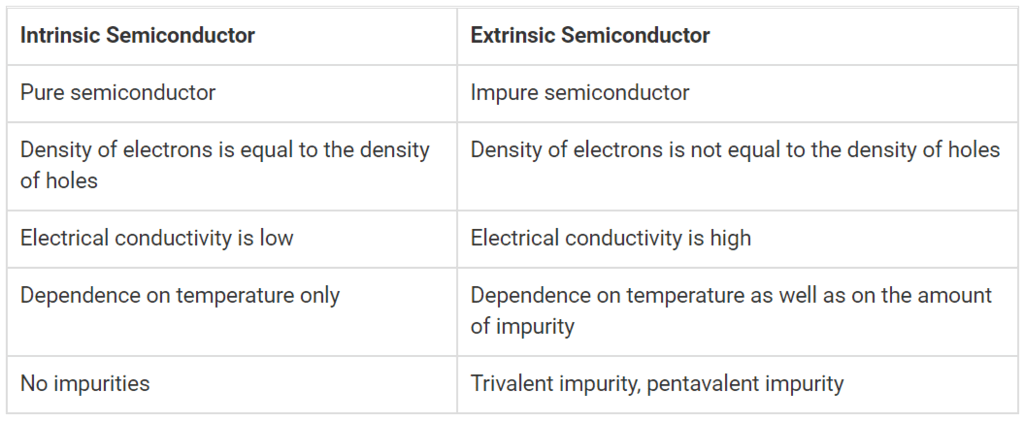The document Semiconductor Types - Notes | Study Analog Electronics - Electrical Engineering (EE) is a part of the Electrical Engineering (EE) Course Analog Electronics.
All you need of Electrical Engineering (EE) at this link: Electrical Engineering (EE)

## Analog Electronics

11 videos|57 docs|108 tests

## Analog Electronics

11 videos|57 docs|108 tests

Track your progress, build streaks, highlight & save important lessons and more!

,

,

,

,

,

,

,

,

,

,

,

,

,

,

,

,

,

,

,

,

,

;